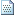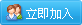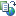web前端

# 常用排序算法之JavaScript实现

### 1、插入排序

1）算法简介

插入排序（Insertion-Sort）的算法描述是一种简单直观的排序算法。它的工作原理是通过构建有序序列，对于未排序数据，在已排序序列中从后向前扫描，找到相应位置并插入。插入排序在实现上，通常采用in-place排序（即只需用到O(1)的额外空间的排序），因而在从后向前扫描过程中，需要反复把已排序元素逐步向后挪位，为最新元素提供插入空间。

2）算法描述和实现

一般来说，插入排序都采用in-place在数组上实现。具体算法描述如下：

1. 从第一个元素开始，该元素可以认为已经被排序；
2. 取出下一个元素，在已经排序的元素序列中从后向前扫描；
3. 如果该元素（已排序）大于新元素，将该元素移到下一位置；
4. 重复步骤3，直到找到已排序的元素小于或者等于新元素的位置；
5. 将新元素插入到该位置后；
6. 重复步骤2~5。

JavaScript代码实现：

```function insertionSort(array) {
if (Object.prototype.toString.call(array).slice(8, -1) === "Array') {
for (var i = 1; i < array.length; i++) {
var key = array[i];
var j = i - 1;
while (j >= 0 && array[j] > key) {
array[j + 1] = array[j];
j--;
}
array[j + 1] = key;
}
return array;
} else {
return 'array is not an Array!';
}
}```

3）算法分析

• 最佳情况：输入数组按升序排列。T(n) = O(n)
• 最坏情况：输入数组按降序排列。T(n) = O(n2)
• 平均情况：T(n) = O(n2)

### 二、二分插入排序

1）算法简介

二分插入（Binary-insert-sort)排序是一种在直接插入排序算法上进行小改动的排序算法。其与直接插入排序算法最大的区别在于查找插入位置时使用的是二分查找的方式，在速度上有一定提升。

2）算法描述和实现

一般来说，插入排序都采用in-place在数组上实现。具体算法描述如下：

1. 从第一个元素开始，该元素可以认为已经被排序；
2. 取出下一个元素，在已经排序的元素序列中二分查找到第一个比它大的数的位置；
3. 将新元素插入到该位置后；
4. 重复上述两步。

JavaScript代码实现：

```function binaryInsertionSort(array) {
if (Object.prototype.toString.call(array).slice(8, -1) === "Array') {
for (var i = 1; i < array.length; i++) {
var key = array[i], left = 0, right = i - 1;
while (left <= right) {
var middle = parseInt((left + right) / 2);
if (key < array[middle]) {
right = middle - 1;
} else {
left = middle + 1;
}
}
for (var j = i - 1; j >= left; j--) {
array[j + 1] = array[j];
}
array[left] = key;
}
return array;
} else {
return 'array is not an Array!';
}
}```

3）算法分析

• 最佳情况：T(n) = O(nlogn)
• 最差情况：T(n) = O(n2)
• 平均情况：T(n) = O(n2)

### 三、选择排序

1）算法简介

选择排序(Selection-sort)是一种简单直观的排序算法。它的工作原理：首先在未排序序列中找到最小（大）元素，存放到排序序列的起始位置，然后，再从剩余未排序元素中继续寻找最小（大）元素，然后放到已排序序列的末尾。以此类推，直到所有元素均排序完毕。

2）算法描述和实现

n个记录的直接选择排序可经过n-1趟直接选择排序得到有序结果。具体算法描述如下：

1. 初始状态：无序区为R[1..n]，有序区为空；
2. 第i趟排序(i=1,2,3...n-1)开始时，当前有序区和无序区分别为R[1..i-1]和R(i..n）。该趟排序从当前无序区中选出关键字最小的记录 R[k]，将它与无序区的第1个记录R交换，使R[1..i]和R[i+1..n)分别变为记录个数增加1个的新有序区和记录个数减少1个的新无序区；
3. n-1趟结束，数组有序化了。

JavaScript代码实现：

```function selectionSort(array) {
if (Object.prototype.toString.call(array).slice(8, -1) === "Array') {
var len = array.length, temp;
for (var i = 0; i < len - 1; i++) {
var min = array[i];
for (var j = i + 1; j < len; j++) {
if (array[j] < min) {
temp = min;
min = array[j];
array[j] = temp;
}
}
array[i] = min;
}
return array;
} else {
return 'array is not an Array!';
}
}```

3）算法分析

• 最佳情况：T(n) = O(n2)
• 最差情况：T(n) = O(n2)
• 平均情况：T(n) = O(n2)

### 四、冒泡排序

1）算法简介

冒泡排序是一种简单的排序算法。它重复地走访过要排序的数列，一次比较两个元素，如果它们的顺序错误就把它们交换过来。走访数列的工作是重复地进行直到没有再需要交换，也就是说该数列已经排序完成。这个算法的名字由来是因为越小的元素会经由交换慢慢“浮”到数列的顶端。

2）算法描述和实现

具体算法描述如下：

1. 比较相邻的元素。如果第一个比第二个大，就交换它们两个；
2. 对每一对相邻元素作同样的工作，从开始第一对到结尾的最后一对，这样在最后的元素应该会是最大的数；
3. 针对所有的元素重复以上的步骤，除了最后一个；
4. 重复步骤1~3，直到排序完成。

JavaScript代码实现：

```function bubbleSort(array) {
if (Object.prototype.toString.call(array).slice(8, -1) === "Array') {
var len = array.length, temp;
for (var i = 0; i < len - 1; i++) {
for (var j = len - 1; j >= i; j--) {
if (array[j] < array[j - 1]) {
temp = array[j];
array[j] = array[j - 1];
array[j - 1] = temp;
}
}
}
return array;
} else {
return 'array is not an Array!';
}
}```

3）算法分析

• 最佳情况：T(n) = O(n)
• 最差情况：T(n) = O(n2)
• 平均情况：T(n) = O(n2)

### 五、快速排序

1）算法简介

快速排序的基本思想：通过一趟排序将待排记录分隔成独立的两部分，其中一部分记录的关键字均比另一部分的关键字小，则可分别对这两部分记录继续进行排序，以达到整个序列有序。

2）算法描述和实现

快速排序使用分治法来把一个串（list）分为两个子串（sub-lists）。具体算法描述如下：

1. 从数列中挑出一个元素，称为 "基准"（pivot）；
2. 重新排序数列，所有元素比基准值小的摆放在基准前面，所有元素比基准值大的摆在基准的后面（相同的数可以到任一边）。在这个分区退出之后，该基准就处于数列的中间位置。这个称为分区（partition）操作；
3. 递归地（recursive）把小于基准值元素的子数列和大于基准值元素的子数列排序。

JavaScript代码实现：

```//方法一
function quickSort(array, left, right) {
if (Object.prototype.toString.call(array).slice(8, -1) === "Array' && typeof left === 'number' && typeof right === 'number') {
if (left < right) {
var x = array[right], i = left - 1, temp;
for (var j = left; j <= right; j++) {
if (array[j] <= x) {
i++;
temp = array[i];
array[i] = array[j];
array[j] = temp;
}
}
quickSort(array, left, i - 1);
quickSort(array, i + 1, right);
};
} else {
return 'array is not an Array or left or right is not a number!';
}
}
var aaa = [3, 5, 2, 9, 1];
quickSort(aaa, 0, aaa.length - 1);
console.log(aaa);

//方法二
var quickSort = function(arr) {
if (arr.length <= 1) { return arr; }
var pivotIndex = Math.floor(arr.length / 2);
var pivot = arr.splice(pivotIndex, 1);
var left = [];
var right = [];
for (var i = 0; i < arr.length; i++){
if (arr[i] < pivot) {
left.push(arr[i]);
} else {
right.push(arr[i]);
}
}
return quickSort(left).concat([pivot], quickSort(right));
};```

3）算法分析

• 最佳情况：T(n) = O(nlogn)
• 最差情况：T(n) = O(n2)
• 平均情况：T(n) = O(nlogn)

### 六、堆排序

1）算法简介

堆排序（Heapsort）是指利用堆这种数据结构所设计的一种排序算法。堆积是一个近似完全二叉树的结构，并同时满足堆积的性质：即子结点的键值或索引总是小于（或者大于）它的父节点。

2）算法描述和实现

具体算法描述如下：

1. 将初始待排序关键字序列(R1,R2....Rn)构建成大顶堆，此堆为初始的无序区；
2. 将堆顶元素R与最后一个元素R[n]交换，此时得到新的无序区(R1,R2,......Rn-1)和新的有序区(Rn),且满足R[1,2...n-1]<=R[n]；
3. 由于交换后新的堆顶R可能违反堆的性质，因此需要对当前无序区(R1,R2,......Rn-1)调整为新堆，然后再次将R与无序区最后一个元素交换，得到新的无序区(R1,R2....Rn-2)和新的有序区(Rn-1,Rn)。不断重复此过程直到有序区的元素个数为n-1，则整个排序过程完成。

JavaScript代码实现：

```/*方法说明：堆排序
@param  array 待排序数组*/
function heapSort(array) {
if (Object.prototype.toString.call(array).slice(8, -1) === "Array') {
//建堆
var heapSize = array.length, temp;
for (var i = Math.floor(heapSize / 2); i >= 0; i--) {
heapify(array, i, heapSize);
}

//堆排序
for (var j = heapSize - 1; j >= 1; j--) {
temp = array;
array = array[j];
array[j] = temp;
heapify(array, 0, --heapSize);
}
} else {
return 'array is not an Array!';
}
}
/*方法说明：维护堆的性质
@param  arr 数组
@param  x   数组下标
@param  len 堆大小*/
function heapify(arr, x, len) {
if (Object.prototype.toString.call(arr).slice(8, -1) === 'Array' && typeof x === 'number') {
var l = 2 * x, r = 2 * x + 1, largest = x, temp;
if (l < len && arr[l] > arr[largest]) {
largest = l;
}
if (r < len && arr[r] > arr[largest]) {
largest = r;
}
if (largest != x) {
temp = arr[x];
arr[x] = arr[largest];
arr[largest] = temp;
heapify(arr, largest, len);
}
} else {
return 'arr is not an Array or x is not a number!';
}
}```

3）算法分析

• 最佳情况：T(n) = O(nlogn)
• 最差情况：T(n) = O(nlogn)
• 平均情况：T(n) = O(nlogn)

### 七、归并排序

1）算法简介

归并排序是建立在归并操作上的一种有效的排序算法。该算法是采用分治法（Divide and Conquer）的一个非常典型的应用。归并排序是一种稳定的排序方法。将已有序的子序列合并，得到完全有序的序列；即先使每个子序列有序，再使子序列段间有序。若将两个有序表合并成一个有序表，称为2-路归并。

2）算法描述和实现

具体算法描述如下：

1. 把长度为n的输入序列分成两个长度为n/2的子序列；
2. 对这两个子序列分别采用归并排序；
3. 将两个排序好的子序列合并成一个最终的排序序列。

JavaScript代码实现：

```function mergeSort(array, p, r) {
if (p < r) {
var q = Math.floor((p + r) / 2);
mergeSort(array, p, q);
mergeSort(array, q + 1, r);
merge(array, p, q, r);
}
}
function merge(array, p, q, r) {
var n1 = q - p + 1, n2 = r - q, left = [], right = [], m = n = 0;
for (var i = 0; i < n1; i++) {
left[i] = array[p + i];
}
for (var j = 0; j < n2; j++) {
right[j] = array[q + 1 + j];
}
left[n1] = right[n2] = Number.MAX_VALUE;
for (var k = p; k <= r; k++) {
if (left[m] <= right[n]) {
array[k] = left[m];
m++;
} else {
array[k] = right[n];
n++;
}
}
}```

3）算法分析

• 最佳情况：T(n) = O(n)
• 最差情况：T(n) = O(nlogn)
• 平均情况：T(n) = O(nlogn)

### 八、桶排序

1）算法简介

桶排序 (Bucket sort)的工作的原理：假设输入数据服从均匀分布，将数据分到有限数量的桶里，每个桶再分别排序（有可能再使用别的排序算法或是以递归方式继续使用桶排序进行排序）。

2）算法描述和实现

具体算法描述如下：

1. 设置一个定量的数组当作空桶；
2. 遍历输入数据，并且把数据一个一个放到对应的桶里去；
3. 对每个不是空的桶进行排序；
4. 从不是空的桶里把排好序的数据拼接起来。

JavaScript代码实现：

```/*方法说明：桶排序
@param  array 数组
@param  num   桶的数量*/
function bucketSort(array, num) {
if (array.length <= 1) {
return array;
}
var len = array.length, buckets = [], result = [], min = max = array, regex = "/^[1-9]+[0-9]*\$/', space, n = 0;
num = num || ((num > 1 && regex.test(num)) ? num : 10);
for (var i = 1; i < len; i++) {
min = min <= array[i] ? min : array[i];
max = max >= array[i] ? max : array[i];
}
space = (max - min + 1) / num;
for (var j = 0; j < len; j++) {
var index = Math.floor((array[j] - min) / space);
if (buckets[index]) {   //  非空桶，插入排序
var k = buckets[index].length - 1;
while (k >= 0 && buckets[index][k] > array[j]) {
buckets[index][k + 1] = buckets[index][k];
k--;
}
buckets[index][k + 1] = array[j];
} else {    //空桶，初始化
buckets[index] = [];
buckets[index].push(array[j]);
}
}
while (n < num) {
result = result.concat(buckets[n]);
n++;
}
return result;
}```

3）算法分析

桶排序最好情况下使用线性时间O(n)，桶排序的时间复杂度，取决与对各个桶之间数据进行排序的时间复杂度，因为其它部分的时间复杂度都为O(n)。很显然，桶划分的越小，各个桶之间的数据越少，排序所用的时间也会越少。但相应的空间消耗就会增大。

### 九、计数排序

1）算法简介

计数排序(Counting sort)是一种稳定的排序算法。计数排序使用一个额外的数组C，其中第i个元素是待排序数组A中值等于i的元素的个数。然后根据数组C来将A中的元素排到正确的位置。它只能对整数进行排序。

2）算法描述和实现

具体算法描述如下：

1. 找出待排序的数组中最大和最小的元素；
2. 统计数组中每个值为i的元素出现的次数，存入数组C的第i项；
3. 对所有的计数累加（从C中的第一个元素开始，每一项和前一项相加）；
4. 反向填充目标数组：将每个元素i放在新数组的第C(i)项，每放一个元素就将C(i)减去1。

JavaScript代码实现：

```function countingSort(array) {
var len = array.length, B = [], C = [], min = max = array;
for (var i = 0; i < len; i++) {
min = min <= array[i] ? min : array[i];
max = max >= array[i] ? max : array[i];
C[array[i]] = C[array[i]] ? C[array[i]] + 1 : 1;
}
for (var j = min; j < max; j++) {
C[j + 1] = (C[j + 1] || 0) + (C[j] || 0);
}
for (var k = len - 1; k >=0; k--) {
B[C[array[k]] - 1] = array[k];
C[array[k]]--;
}
return B;
}```

3）算法分析

当输入的元素是n 个0到k之间的整数时，它的运行时间是 O(n + k)。计数排序不是比较排序，排序的速度快于任何比较排序算法。由于用来计数的数组C的长度取决于待排序数组中数据的范围（等于待排序数组的最大值与最小值的差加上1），这使得计数排序对于数据范围很大的数组，需要大量时间和内存。

[本日志由 admin 于 2014-09-02 08:44 AM 编辑]文章来自: 本站原创Tags: